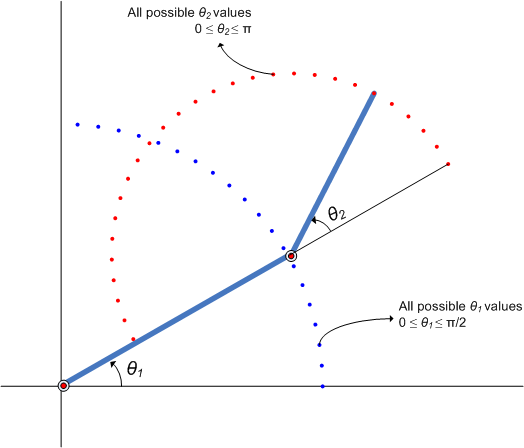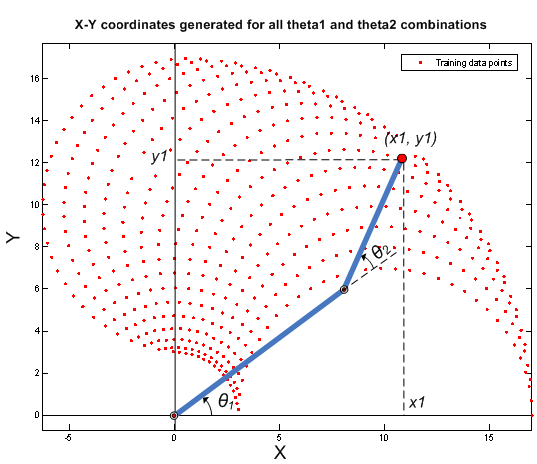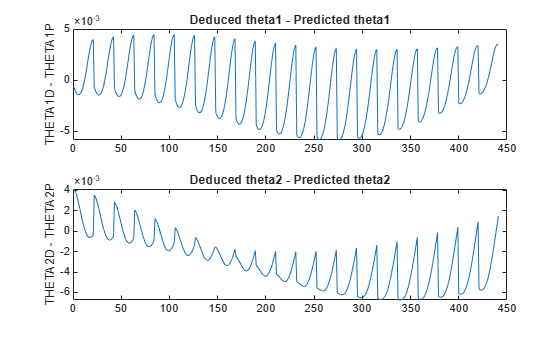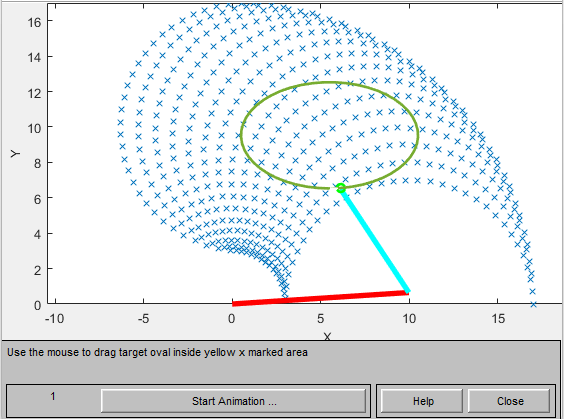# Modeling Inverse Kinematics in a Robotic Arm

This example shows how to use a fuzzy system to model the inverse kinematics in a two-joint robotic arm.

### What Is Inverse Kinematics?

Kinematics is the science of motion. In a two-joint robotic arm, given the angles of the joints, the kinematics equations give the location of the tip of the arm. Inverse kinematics refers to the reverse process. Given a desired location for the tip of the robotic arm, what should the angles of the joints be so as to locate the tip of the arm at the desired location. There is usually more than one solution and can at times be a difficult problem to solve.

This is a typical problem in robotics that needs to be solved to control a robotic arm to perform tasks it is designated to do. In a 2-dimensional input space, with a two-joint robotic arm and given the desired coordinate, the problem reduces to finding the two angles involved. The first angle is between the first arm and the ground (or whatever it is attached to). The second angle is between the first arm and the second arm.Figure 1: Illustration showing the two-joint robotic arm with the two angles, `theta1` and `theta2`

### Why Use Fuzzy Logic?

For simple structures like the two-joint robotic arm, it is possible to mathematically deduce the angles at the joints given the desired location of the tip of the arm. However with more complex structures (for example: n-joint robotic arms operating in a 3-dimensional input space) deducing a mathematical solution for the inverse kinematics may prove challenging.

Using fuzzy logic, we can construct a fuzzy inference system that deduces the inverse kinematics if the forward kinematics of the problem is known, hence sidestepping the need to develop an analytical solution. Also, the fuzzy solution is easily understandable and does not require special background knowledge to comprehend and evaluate it.

In the following section, a broad outline for developing such a solution is described, and later, the detailed steps are elaborated.

### Overview of Fuzzy Solution

Since the forward kinematics formulae for the two-joint robotic arm are known, x and y coordinates of the tip of the arm are deduced for the entire range of angles of rotation of the two joints. The coordinates and the angles are saved to be used as training data to train an ANFIS (adaptive neuro-fuzzy inference system) network.

During training, the ANFIS network learns to map the coordinates (`x`, `y`) to the angles (`theta1`, `theta2`). The trained ANFIS network is then used as a part of a larger control system to control the robotic arm. Knowing the desired location of the robotic arm, the control system uses the trained ANFIS network to deduce the angular positions of the joints and applies force to the joints of the robotic arm accordingly to move it to the desired location.

### What Is ANFIS?

ANFIS stands for adaptive neuro-fuzzy inference system. It is a hybrid neuro-fuzzy technique that brings learning capabilities of neural networks to fuzzy inference systems. The learning algorithm tunes the membership functions of a Sugeno-type fuzzy inference system using the training input/output data.

In this case, the input/output data refers to the "coordinates/angles" dataset. The coordinates act as input to the ANFIS and the angles act as the output. The learning algorithm teaches the ANFIS to map the coordinates to the angles through a process called training. At the end of training, the trained ANFIS network would have learned the input-output map and be ready to be deployed into the larger control system solution.

### Data Generation

Let `theta1` be the angle between the first arm and the ground. Let `theta2` be the angle between the second arm and the first arm (Refer to Figure 1 for illustration). Let the length of the first arm be `l1` and that of the second arm be `l2`.

Assume that the first joint has limited freedom to rotate and it can rotate between 0 and 90 degrees. Similarly, assume that the second joint has limited freedom to rotate and can rotate between 0 and 180 degrees. (This assumption takes away the need to handle some special cases which will confuse the discourse.) Hence, `0<=theta1<=pi/2` and `0<=theta2<=pi`.Figure 2: Illustration showing all possible `theta1` and `theta2` values.

Now, for every combination of `theta1` and `theta2` values the x and y coordinates are deduced using forward kinematics formulae.

The following code snippet shows how data is generated for all combination of `theta1` and `theta2` values and saved into a matrix to be used as training data. The reason for saving the data in two matrices is explained in the following section.

```l1 = 10; % length of first arm l2 = 7; % length of second arm theta1 = 0:0.1:pi/2; % all possible theta1 values theta2 = 0:0.1:pi; % all possible theta2 values [THETA1,THETA2] = meshgrid(theta1,theta2); % generate grid of angle values X = l1 * cos(THETA1) + l2 * cos(THETA1 + THETA2); % compute x coordinates Y = l1 * sin(THETA1) + l2 * sin(THETA1 + THETA2); % compute y coordinates data1 = [X(:) Y(:) THETA1(:)]; % create x-y-theta1 dataset data2 = [X(:) Y(:) THETA2(:)]; % create x-y-theta2 dataset```

The following plot shows all the X-Y data points generated by cycling through different combinations of `theta1` and `theta2` and deducing x and y coordinates for each. The plot can be generated by using the following code. The plot is annotated further for easier understanding.

``` plot(X(:),Y(:),'r.'); axis equal; xlabel('X','fontsize',10) ylabel('Y','fontsize',10) title('X-Y coordinates for all theta1 and theta2 combinations','fontsize',10) ```Figure 3: X-Y coordinates generated for all `theta1` and `theta2` combinations using forward kinematics formulae

### Building ANFIS Networks

One approach to building an ANFIS solution for this problem, is to build two ANFIS networks, one to predict `theta1` and the other to predict `theta2`.

In order for the ANFIS networks to be able to predict the angles they have to be trained with sample input-output data. The first ANFIS network will be trained with X and Y coordinates as input and corresponding `theta1` values as output. The matrix `data1` contains the `x-y-theta1` dataset required to train the first ANFIS network. Therefore `data1` will be used as the dataset to train the first ANFIS network.

Similarly, the second ANFIS network will be trained with X and Y coordinates as input and corresponding `theta2` values as output. The matrix `data2` contains the `x-y-theta2` dataset required to train the second ANFIS network. Therefore `data2` will be used as the dataset to train the second ANFIS network.

To train an ANFIS network, first specify the training options using the `anfisOptions` command. For this example, specify an FIS object with `7` membership functions for each input variable. Train the system for `150` epochs and suppress the Command Window display of training information.

```opt = anfisOptions; opt.InitialFIS = 7; opt.EpochNumber = 150; opt.DisplayANFISInformation = 0; opt.DisplayErrorValues = 0; opt.DisplayStepSize = 0; opt.DisplayFinalResults = 0;```

Train an ANFIS system using the first set of training data, `data1`.

`disp('--> Training first ANFIS network.')`
```--> Training first ANFIS network. ```
`anfis1 = anfis(data1,opt);`

Change the number of input membership functions and train an ANFIS system using the second set of training data, `data2`.

`disp('--> Training second ANFIS network.')`
```--> Training second ANFIS network. ```
```opt.InitialFIS = 6; anfis2 = anfis(data2,opt);```

For this example, the number of input membership functions and training epochs were selected based on experimentation with different potential values.

`anfis1` and `anfis2` represent the two trained ANFIS networks that will be deployed in the larger control system.

Once the training is complete, the two ANFIS networks have learned to approximate the angles (`theta1`, `theta2`) as a function of the coordinates (`x`, `y`). One advantage of using the fuzzy approach is that the ANFIS network can now approximate the angles for coordinates that are similar but not exactly the same as it was trained with. For example, the trained ANFIS networks are now capable of approximating the angles for coordinates that lie between two points that were included in the training dataset. This will allow the final controller to move the arm smoothly in the input space.

We now have two trained ANFIS networks which are ready to be deployed into the larger system that will utilize these networks to control the robotic arms.

### Validating ANFIS Networks

Having trained the networks, an important follow up step is to validate the networks to determine how well the ANFIS networks would perform inside the larger control system.

Since this example problem deals with a two-joint robotic arm whose inverse kinematics formulae can be derived, it is possible to test the answers that the ANFIS networks produce with the answers from the derived formulae.

Assume that it is important for the ANFIS networks to have low errors within the operating range `0<x<2` and `8<y<10`.

```x = 0:0.1:2; % x coordinates for validation y = 8:0.1:10; % y coordinates for validation```

The `theta1` and `theta2` values are deduced mathematically from the x and y coordinates using inverse kinematics formulae.

```[X,Y] = meshgrid(x,y); c2 = (X.^2 + Y.^2 - l1^2 - l2^2)/(2*l1*l2); s2 = sqrt(1 - c2.^2); THETA2D = atan2(s2,c2); % theta2 is deduced k1 = l1 + l2.*c2; k2 = l2*s2; THETA1D = atan2(Y,X) - atan2(k2,k1); % theta1 is deduced```

`THETA1D` and `THETA2D` are the variables that hold the values of `theta1` and `theta2` deduced using the inverse kinematics formulae.

`theta1` and `theta2` values predicted by the trained ANFIS networks are obtained by using the command `evalfis` which evaluates a FIS for the given inputs.

Here, `evalfis` is used to find out the FIS outputs for the same x-y values used earlier in the inverse kinematics formulae.

```XY = [X(:) Y(:)]; THETA1P = evalfis(anfis1,XY); % theta1 predicted by anfis1 THETA2P = evalfis(anfis2,XY); % theta2 predicted by anfis2```

Now, we can see how close the FIS outputs are with respect to the deduced values.

```theta1diff = THETA1D(:) - THETA1P; theta2diff = THETA2D(:) - THETA2P; subplot(2,1,1) plot(theta1diff) ylabel('THETA1D - THETA1P') title('Deduced theta1 - Predicted theta1') subplot(2,1,2) plot(theta2diff) ylabel('THETA2D - THETA2P') title('Deduced theta2 - Predicted theta2')```The errors are in the `1e-3` range which is a fairly good number for the application it is being used in. However this may not be acceptable for another application, in which case the parameters to the `anfis` function may be tweaked until an acceptable solution is arrived at. Also, other techniques like input selection and alternate ways to model the problem may be explored.

### Building a Solution Around the Trained ANFIS Networks

Now given a specific task, such as robots picking up an object in an assembly line, the larger control system will use the trained ANFIS networks as a reference, much like a lookup table, to determine what the angles of the arms must be, given a desired location for the tip of the arm. Knowing the desired angles and the current angles of the joints, the system will apply force appropriately on the joints of the arms to move them towards the desired location.

The `invkine` command launches a GUI that shows how the two trained ANFIS networks perform when asked to trace an ellipse.Figure 4: GUI for Inverse Kinematics Modeling.

The two ANFIS networks used in the example have been pretrained and are deployed into a larger system that controls the tip of the two-joint robot arm to trace an ellipse in the input space.

The ellipse to be traced can be moved around. Move the ellipse to a slightly different location and observe how the system responds by moving the tip of the robotic arm from its current location to the closest point on the new location of the ellipse. Also observe that the system responds smoothly as long as the ellipse to be traced lies within the 'x' marked spots which represent the data grid that was used to train the networks. Once the ellipse is moved outside the range of data it was trained with, the ANFIS networks respond unpredictably. This emphasizes the importance of having relevant and representative data for training. Data must be generated based on the expected range of operation to avoid such unpredictability and instability issues.